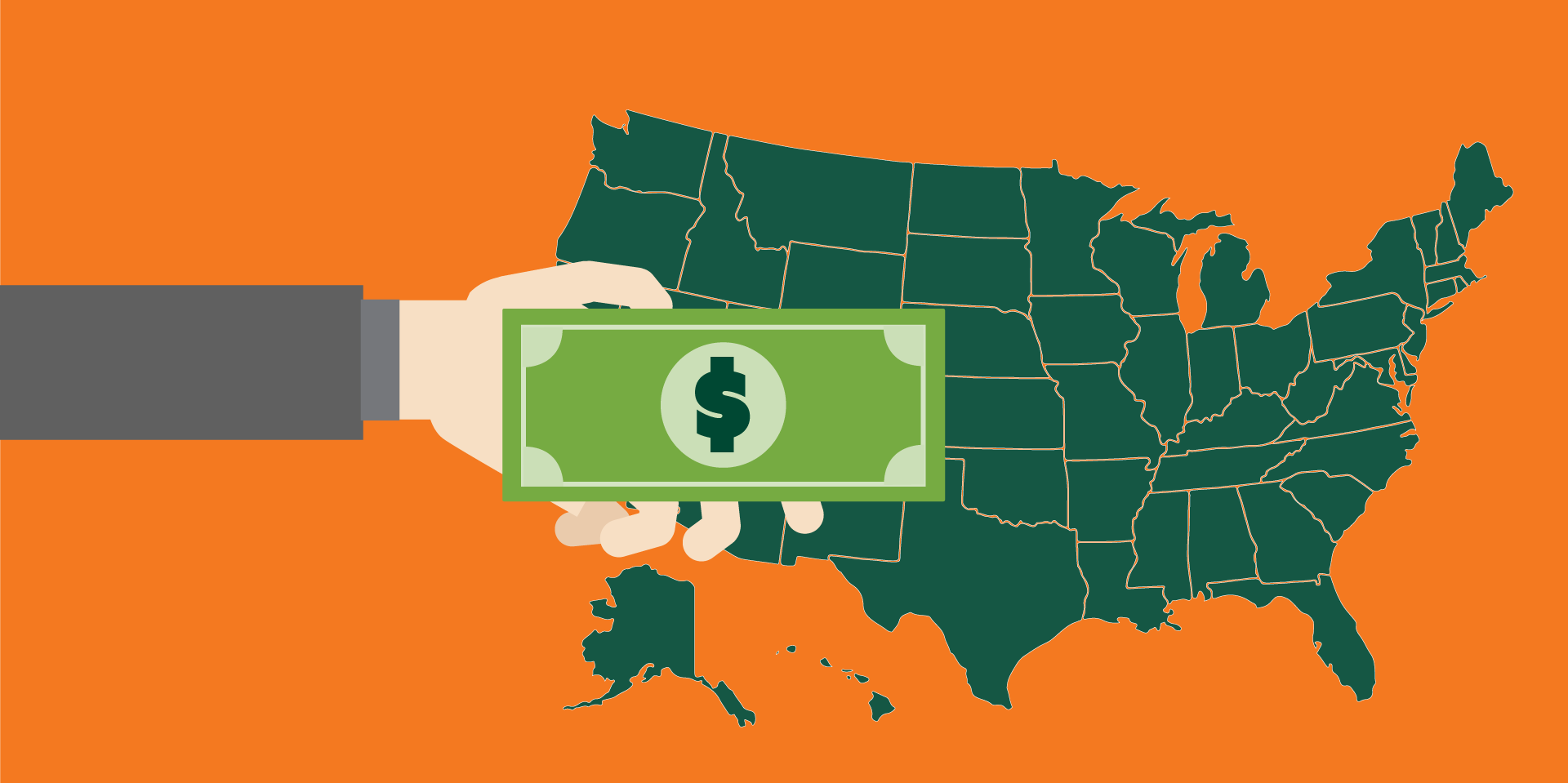## CPS Fee Structure 2018-2020

CPS – Sri Lanka follows an affordable, simple, and transparent fee structure across all its faculties and programs. Fee structure is credit based and is uniform across all faculties and programs.## Rs. 85,000.00

Payable entirely in advance at the time of registration

Breakup as follows:

• Registration: Rs 2500
• Examination: Rs 350 X 50 = Rs 17,500
• Tuition: Rs 1000 X 50 = Rs 50,000

## Rs. 173,000.00

Payable entirely in advance at the time of registration

Breakup as follows:

• Registration: Rs 5000
• Examination: Rs 350 X 80 = Rs 28,000
• Tuition: Rs 1500 X 80 = Rs 120,000

## Rs. 245,000.00

Payable entirely in advance at the time of registration

Breakup as follows:

• Registration: Rs 5000
• Examination: Rs 350 X 100 = Rs 35,000
• Tuition: Rs 1800 X 100 = Rs 180,000

## Rs. 419,000.00

Payable in four years; (First year: Rs. 104,750; Second Year: Rs. 104,750; Third Year: Rs. 104,750; Forth Year: Rs. 104,750)

Breakup as follows:

• Registration: Rs 5000 X 04 = Rs 20,000 (Payable Rs 5000 per annum)
• Administration: Rs 5000 X 04 = Rs 20,000 (Payable Rs 5000 per annum)
• Examination: Rs 350 X 120 = Rs 42,000 (Payable Rs 10,500 per annum)
• Tuition: Rs 2600 X 120 = Rs 312,000 (Payable Rs 78,000 per annum)

## Rs. 809,000.00

Payable in four years; (First year: Rs 166,000; Second Year: Rs 166,000; Third Year: Rs 166,000; Forth Year: Rs 145,000)

Breakup as follows:

• Registration: Rs 5000 X 04 = Rs 20,000 (Payable Rs 5000 per annum)
• Administration: Rs 5000 X 04 = Rs 20,000 (Payable Rs 5000 per annum)
• Examination: Rs 1200 X 120 = Rs 144,000 (Payable Rs 36,000 per annum)
• Tuition: Rs 5000 X 120 = Rs 600,000 (Payable Rs 120,000 per annum)

## Rs. 807,000.00

Payable in five years; (First year: Rs 156,400; Second Year: Rs 156,400; Third Year: Rs 156,400; Forth Year: Rs 156,400, Fifth Year: Rs 181,400)

Breakup as follows:

• Registration: Rs 5000 X 05 = Rs 25,000 (Payable Rs 5000 per annum)
• Administration: Rs 5000 X 05 = Rs 25,000 (Payable Rs 5000 per annum)
• Examination: Rs 1100 X 120 = Rs 132,000 (Payable Rs 26,400 per annum)
• Tuition: Rs 5000 X 120 = Rs 600,000 (Payable Rs 120,000 per annum)

## Rs. 865,500.00

Payable in two years; (First year: Rs 415,250; Second Year: Rs 450,250)

Breakup as follows:

• Registration: Rs 15000 X 02 = Rs 30,000 (Payable Rs 15,000 per annum)
• Administration: Rs 15000 X 02 = Rs 30,000 (Payable Rs 15,000 per annum)
• Examination: Rs 350 X 350 = Rs 122,500 (Payable Rs 61,250 per annum)
• Tuition: Rs 3600 X 180 = Rs 648,000 (Payable Rs 324,000 per annum)

## Rs. 1,190,000.00

Payable in two years; (First year: Rs 570,000; Second Year: 620,000)

Breakup as follows:

• Registration: Rs 50,000 X 02 = Rs 100,000 (Payable Rs 50,000 per annum)
• Administration: Rs 20,000 X 02 = Rs 40,000 (Payable Rs 20,000 per annum)
• Research Evaluation: Rs 500 X 400 = Rs 200,000 (Payable Rs 100,000 per annum)
• Research Supervision: Rs 2000 X 400 = Rs 800,000 (Payable Rs 400,000 per annum)

## Rs. 1,190,000.00

Payable in two years; (First year: Rs 700,000; Second Year: Rs 490,000)

Breakup as follows:

• Registration: Rs 50,000 X 02 = Rs 100,000 (Payable Rs 50,000 per annum)
• Administration: Rs 20000 X 02 = Rs 40000 (Payable Rs 20,000 per annum)
• Research Evaluation: Rs 500 X 400 = Rs 200,000 (Payable Rs 100,000 per annum)
• Research Supervision: Rs 2000 X 400 = Rs 800,000 (Payable Rs 400,000 per annum)

## Rs. 870,000.00

Payable entirely in advance at the time of registration

Breakup as follows:

• Registration: Rs 50,000